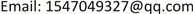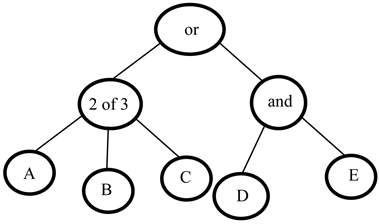﻿ 支持访问策略隐藏的属性基加密方案研究 Research of CP-ABE Supported Hidden Access Policy

Computer Science and Application
Vol. 09  No. 02 ( 2019 ), Article ID: 28804 , 7 pages
10.12677/CSA.2019.92032

Research of CP-ABE Supported Hidden Access Policy

Xinglan Zhang, Mingming Wang

Beijing University of Technology, BeijingReceived: Jan. 16th, 2019; accepted: Jan. 28th, 2019; published: Feb. 12th, 2019ABSTRACT

Attribute-based encryption that supports policy hiding not only protects plaintext, but also protects user’s sensitive information. The existing schemes have limitations on the access structure and weak strategy expression. This paper proposes an attribute-based encryption scheme for hidden access policies. It implements policy hiding by transforming the access structure into a property list and then encrypting the property list. The scheme has no restrictions on the access structure. In addition, outsourcing partial decryption calculations reduce the computational burden on users. Analysis shows that the new scheme enhances the flexibility of policy expression and decryption efficiency while implementing policy hiding, and proves to be secure under the DBDH assumption.

Keywords:CP-ABE, Hidden Policy, DBDH Assumption1. 引言

2. 预备知识

2.1. 双线性映射

1) 双线性性质： $e\left({g}^{a},{h}^{b}\right)=e{\left(g,h\right)}^{ab}$。g，h是G1中的元素，a，b是 ${Z}_{p}^{*}$ 中的元素。

2) 非退化性： $e\left(g,g\right)\ne 1$

3) 可计算性：对G1中的所有元素 $g,h$，存在一个有效的算法计算出 $e\left(g,h\right)$ 的值。

2.2. DBDH假设

2.3. 本文方案

2.3.1. 系统模型

1) 可信机构：可信机构是完全受信任的中央授权机构，它生成系统的公共参数并为用户计算私钥。

2) 数据拥有者：数据拥有者的工作是制定访问结构、对数据加密并将密文上传到云服务器。

3) 用户：用户从云端服务器下载密文，然后向可信机构提交自己的属性列表，并从可信机构获得相应的私钥，当且仅当属性列表满足访问结构的时候可以成功解密密文。

4) 云服务器：云服务器负责存储密文和解密外包计算。云服务器是不完全受信任的，它有可能会泄露存储的数据或给用户故意返回错误的计算结果。

2.3.2. 访问结构转换规则

1) 如果节点是and，则 ${W}_{\alpha }=\left\{{W}_{1}=\left[{a}_{1},{a}_{2}\cdots {a}_{\alpha k}\right]\right\}$

2) 如果节点为or，则 ${W}_{\alpha }=\left\{{W}_{1}=\left[{a}_{1}\right],{W}_{2}=\left[{a}_{2}\right]\cdots {W}_{\alpha k}=\left[{a}_{\alpha k}\right]\right\}$

3) 如果节点为of，门限值为h，则 ${W}_{\alpha }=\left\{{W}_{i}=\left[{a}_{i}\cdots {a}_{m}\right]\cdots {W}_{n}=\left[{a}_{i}\cdots {a}_{m}\right]\right\}\left(n={C}_{{\alpha }_{k}}^{h}\right)$

2.3.3. 访问结构

2.4. 方案安全模型Figure 1. Access tree T

3. 算法设计

1) 初始化过程( 1 λ )

2) 加密过程(PK, T, M)

3) 提取私钥

4) 外包解密

$C{T}^{\prime }=\underset{i=1}{\overset{m}{\prod }}e\left({C}_{i,t},{D}_{i}\right)=e{\left(g,g\right)}^{\underset{i=1}{\overset{m}{\sum }}s{a}_{i,t}·{d}_{i}/\theta {a}_{i,t}}=e{\left(g,g\right)}^{s/\theta ·\underset{i=1}{\overset{m}{\sum }}{d}_{i}}=e{\left(g,g\right)}^{sd/\theta }$

5) 用户解密

$\frac{C}{e\left({C}_{0},{D}_{0}\right)•C{{T}^{\prime }}^{\theta }}=\frac{M{Y}^{s}}{e\left({g}^{s},{g}^{y-d}\right)•e{\left(g,g\right)}^{\left(sd/\theta \right)\theta }}=\frac{Me{\left(g,g\right)}^{ys}}{e{\left(g,g\right)}^{sy-sd}•e{\left(g,g\right)}^{sd}}=M$；否则，用户无权解密。

4. 安全性证明

$\mu =0$ 时，敌手A获得有效密文 $C{T}^{\prime }$。敌手的优势为： $\mathrm{Pr}\left[j\prime =j|Z=e{\left(g,g\right)}^{abc}\right]=\frac{1}{2}+\epsilon$。当 $\mu =\text{1}$ 时，敌手获得的密文 $C{T}^{\prime }$ 是随机的，无法得知它明文的任何信息， $\mathrm{Pr}\left[{j}^{\prime }\ne j|Z=e{\left(g,g\right)}^{z}\right]=\frac{1}{2}$。因此，模拟器在解决DBDH假设上总的优势为： $\frac{1}{2}\mathrm{Pr}\left[{j}^{\prime }=j|Z=e{\left(g,g\right)}^{abc}\right]+\frac{1}{2}\mathrm{Pr}\left[{j}^{\prime }\ne j|\text{}Z=\text{}e{\left(g,g\right)}^{z}\right]-\frac{1}{2}=\frac{\epsilon }{2}$

5. 效率分析Table 1. Comparison of this scheme and other schemes

6. 结束语

Research of CP-ABE Supported Hidden Access Policy[J]. 计算机科学与应用, 2019, 09(02): 275-281. https://doi.org/10.12677/CSA.2019.92032

1. 1. 曹珍富, 董晓蕾, 周俊, 等. 大数据安全与隐私保护研究进展[J]. 计算机研究与发展, 2016, 53(10): 2137-2151.

2. 2. Shamir, A. (1985) Identity-Based Cryptosystems and Signature Schemes. In: Advances in Cryptology, Springer, Berlin Heidelberg, 47-53. https://doi.org/10.1007/3-540-39568-7_5

3. 3. Sahai, A. and Waters, B. (2005) Fuzzy Identity-Based Encryption. Advances in Cryptology—EUROCRYPT 2005. Springer, Berlin Heidelberg, 457-473. https://doi.org/10.1007/11426639_27

4. 4. Chander, V.P., Babu, G.C., Babu, S.S., et al. (2013) Procure Data Storage in Clode Using CPABE. International Journal of Computer Trends & Technology, 4, 77-84.

5. 5. Goyal, V., Pandey, O., Sahai, A., et al. (2006) Attribute-Based Encryption for Fine-Grained Access Control of Encrypted Data. Proceedings of ACMCCS’06, ACM Press, New York, 89-98.

6. 6. 冯登国, 陈成. 属性密码学研究[J]. 密码学报, 2014, 1(1): 1-12.

7. 7. 苏金树, 曹丹, 王小峰. 属性基加密机制[J]. 软件学报, 2011, 22(6): 1299-1315.

8. 8. Nishide, T., Yoneyama, K. and Ohta, K. (2008) Attribute-Based Encryption with Partially Hidden Encryptor-Specified Access Structures. ACNS 2008: Applied Cryptography and Network Security, New York, 3-6 June 2008, 111-129. https://doi.org/10.1007/978-3-540-68914-0_7

9. 9. Lai, J., Deng, R.H. and Li, Y. (2011) Fully Secure Cipertext-Policy Hiding CP-ABE. ISPEC 2011: Information Security Practice and Experience, Guangzhou, May 30-June 1 2011, 24-39. https://doi.org/10.1007/978-3-642-21031-0_3

10. 10. Hur, J. (2013) Attribute-Based Secure Data Sharing with Hidden Policies in Smart Grid. IEEE Transactions on Parallel and Distributed Systems, 24, 2171-2180. https://doi.org/10.1109/TPDS.2012.61

11. 11. 杜瑞颖, 沈剑, 陈晶, 周顺淦. 基于策略隐藏属性加密的云访问控制方案[J]. 武汉大学学报(理学版), 2016, 62(3): 242-248.

12. 12. 宋衍, 韩臻, 刘凤梅, 等. 基于访问树的策略隐藏属性加密方案[J]. 通信学报, 2015, 36(9): 119-126.

13. 13. Green, M., Hohenberger, S. and Waters, B. (2011) Outsourcing the Decryption of ABE Ciphertexts. Proceedings of the 20th USENIX Conference on Security, San Francisco, 8-12 August 2011, 34-34.

14. 14. Lai, J., Deng, R.H., Guan, C., et al. (2015) Attribute-Based Encryption with Verifiable Outsourced Decryption. IEEE Transactions on Information Forensics & Security, 10, 1384-1393. https://doi.org/10.1109/TIFS.2015.2410137

15. 15. Liu, H., Wang, X. and Zhang, P. (2015) Verifying Outsourced Decryption of CP-ABE with Signature. 2015 4th International Conference on Mechatronics, Materials, Chemistry and Computer En-gineering.

16. 16. 马华, 白翠翠, 李宾, 刘振华. 支持属性撤销和解密外包的属性基加密方案[J]. 西安电子科技大学学报, 2015, 42(6): 6-10+55.

17. 17. 丁晓红, 秦敬源, 王新. 一种属性基加密方案的外包解密方法[J]. 计算机科学, 2016, 43(S1): 357-360.

18. 18. Emura, K., Miyaji, A., Nomura, A., et al. (2009) A Ciphertext-Policy Attribute-Based Encryption Scheme with Con-stant Ciphertext Length. Proceedings of ISPEC 2009, Springer, Berlin, 12-23. https://doi.org/10.1007/978-3-642-00843-6_2